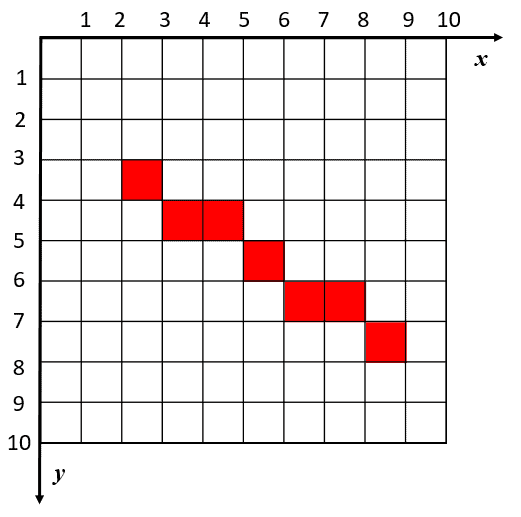## 1. Overview

In this tutorial, we’ll review the Bresemhan’s line algorithm, a widely used computer graphics algorithm for drawing lines on a display device. We provide the mathematical description and the pseudocode of the algorithm. Finally, we show a numerical example.

## 2. History

Bresenham’s line algorithm was first introduced by Jack Elton Bresenham in 1965. Bresenham was working at IBM’s Watson Research Center at the time, where he developed the algorithm as a way to efficiently draw lines on a cathode ray tube display device. Other researchers later refined and improved the algorithm, and today it is a standard part of many computer graphics and image processing libraries.

## 3. Description

Bresenham’s line algorithm is a simple and efficient algorithm for drawing lines on an image. It uses only integer addition, subtraction, and bit shifting. In other words, only very cheap operations.

The algorithm is based on the observation that the slope of a line between two points can be expressed as a ratio of the change in the-coordinate to the change in the-coordinate. This ratio is known as the slope of the line.

Bresenham’s line algorithm works by incrementally plotting the pixels that are closest to the line, one at a time. The algorithm computes the coordinates of the successive points by incrementing theandcoordinates. An error term is computed at each step to determine iforshould be incremented. Hence, the line can be drawn without expensive division or multiplication operations. By repeating a simple process the algorithm can draw a smooth and accurate line.

Extended versions of Bresenham’s algorithm allow drawing ellipses, circles, and Bezier curves.

## 4. Pseudocode

Here’s the pseudocode of Bresenham’s line algorithm:First, the increment variablesandare initialized according to the direction of the line. The variableis a measurement for the deviation of the current pixel from the line. Such error computation is used to guess the next pixel. At each step, at least one coordinate (or) is incremented by +1 or -1 depending on the current error value.

The functionis called at each step to color the pixel of coordinates. The process is terminated when the current point matches the endpoint.

The time complexity of the algorithm is, and the space complexity is.

## 5. Example

Now let’s draw a segment passing through the points,by using the Bresenham algorithm.

First, we compute the horizontal and vertical projections of the line segment:and the corresponding initial error value:In this case, the increment values of the coordinates areand. Here’s the set of points generated by the algorithm and the corresponding error values:Finally, we draw the resulting line on an image of sizepixels:## 6. Conclusion

In this article, we described Bresemhan’s line algorithm, a simple and efficient algorithm for drawing lines on an image. We provided the pseudocode of the algorithm and a numerical example.